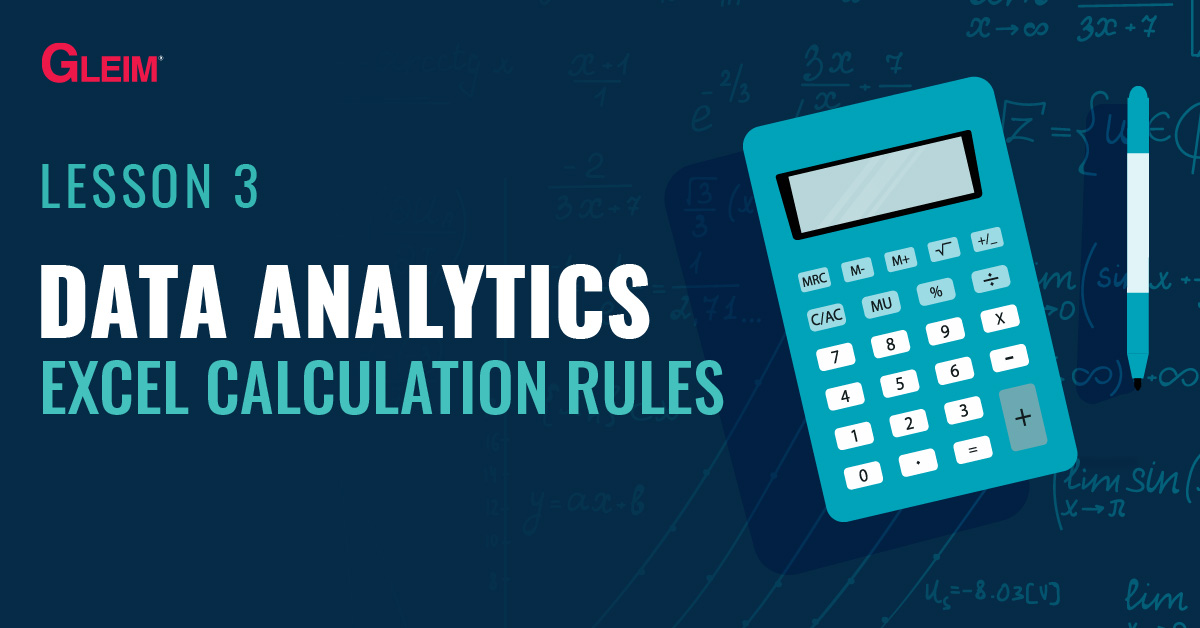# Data Analytics 3: Excel Calculation RulesCalculations in Excel are performed through formulas. For example, to add up numbers A and B, we use the formula A + B. The rules governing formulas in Excel will be discussed in this blog.

## Syntax

The “syntax” for Excel is the set of rules that govern the structures of the formulas. Without correct syntax, the calculation cannot be performed (i.e., it is not executable).

Here is a list of syntax fundamentals for creating formulas in Excel:

1. The formula should generally be entered into a specific cell (e.g., A1).
2. All formulas start with an equal sign = . For example, to use a formula to calculate 10 + 20 in cell A1, we must enter the formula as = 10 + 20 .
3. The calculation result is stored and displayed in the cell where we enter the formula. For example, if we enter the formula = 10 + 20 in cell A1, cell A1 stores and displays the result 30.
4. The content in a cell is regarded as one formula. For example, if we enter = 10*2 + 20/4 in cell A1, the whole content in cell A1 is treated as one formula.
5. Cell references can be used in formulas. (For more details about cell references, see Cell References.)

## Logic

In addition to correct syntax, an Excel formula must follow certain logical rules. Without correct logic, the calculation can be performed but the formula may not return the desired result (i.e., it is executable but may be incorrect).

Below is the order of mathematical operations:

Order of OperationOperator
1stExcel Functions
2nd( )
3rd^
4th* /
5th+ –

1. Multiplication and division must be performed before addition and subtraction.

Example 1:
For the formula = 1 + 2 * 3, multiplication must be done first. The calculation result is 7. You would multiply 2 × 3 to get 6 and then add the 1 (1 + 6).

Example 2:
For the formula = 20 / 4 + 10 * 2, both multiplication and division must be done first. The calculation result is 25. You would divide 20 by 4 to get 5 and then multiple 10 × 2 to get 20 (5 + 20).

2. Exponents (powers) are calculated before multiplication and division.

Example:
For the formula = 1 + 2 * 3 ^ 4, the exponent is calculated first. The result of 81 (3 to the power of 4) is multiplied by 2 to get 162. The final result is 163 (1 + 162).

3. Calculations inside parentheses are done before all mathematical operators.

Example 1:
For the formula = (1 + 2) ^ 3 + 4, the calculation inside the parenthesis is done first to get the result of 3. The exponent is then computed to yield the result 27. The final result is 31 (27 + 4).

Example 2:
For the formula = (((1 + 2) * 3 – 4) ^ 2 + 5) * 6, the calculation in the innermost parenthesis is done first to yield the result of 3 (1 + 2). The calculation in the middle set of parenthesis is then performed to yield the result of 5 (3 × 3 – 4), keeping in mind the multiplication is done first. The calculation in the outermost parenthesis is done next, yielding the result of 30 (5^2 + 5). The final result is 180 (30 × 6).

4. Excel functions are calculated before parenthesis.

Example:
For the formula = (sum(1,2,3,4) + 5)^2, the sumfunction is executed first to get the result of 10 (1+2+3+4). The calculation in the parenthesis is then computed to yield the result of 15 (10 + 5). The final result is 225 (15 ^ 2). (For more details about Excel functions, see Excel Functions.)

## Datetime and Text Data

Datetime data are treated as numerical values in Excel, with any difference in day altered by the number one. The syntax and logic of formulas used to calculate numerical values also applies to datetime data.
For example, the formula = “2020/1/1” + 1 adds one day to the date January 1, 2020. The result returned is January 2, 2020.

Text data, on the other hand, are treated as a string of individual characters. For example, the text “Excel” is a string of the character “E”, followed by the characters “x”, “c”, “e”, and “l”. Even if the text entry is in the form of a number, such as “20001”, the entry is treated as the character “2”, followed by three characters “0”, and one character “1” rather than the numerical value 20001. Therefore, the basic numerical operations such as addition, subtraction, multiplication, and division do not apply to text data.

## Learning Excel Data Analytics with Gleim

At Gleim, we know learning data analytics is vital for future CPAs, CMAs, and CIAs. That is why we are offering this series of data analytics blogs and are continually updating all of our CPA, CMA, and CIA Review materials with the necessary information you need to pass your exams.

If you’re ready to get video tutorials specifically for data analytics in the CPA Exam and have more data to practice with syntaxes and logics of Excel formulas, look no further than the Gleim Premium CPA Review.

Python Lessons
Python Basics
Conditional Statements
Loops
Functions and Modules
Numerical Python (NumPy)
Pandas Basics
Pandas Data Capture and Cleansing
Merging and Grouping
Manipulating Text and Datetime Data
Visualization
Web Scraping
Errors and Exceptions